• 1.对偶问题 随着线性规划应用的逐步深入，人们发现一个线性规划问题往往伴随着与之配对的、两者有着密切的联系的另一个线性规划问题，将其中一个称为原问题，另外一个称之为对偶问题。 对偶理论深刻揭示了原问题与...
1.对偶问题
随着线性规划应用的逐步深入，人们发现一个线性规划问题往往伴随着与之配对的、两者有着密切的联系的另一个线性规划问题，将其中一个称为原问题，另外一个称之为对偶问题。对偶理论深刻揭示了原问题与对偶问题的内在联系，由对偶问题引申出来的对偶解有着重要经济意义，是经济学中重要的概念工具之一，对偶理论充分显示线性规划理论逻辑上的严谨性与结构上的对称性，它是线性规划的重要成果。
原问题的例子
某家具厂木器车间生产木门和木窗的两种产品，加工木门收入56\扇，加工木窗收入30\扇，生产一扇木盟需要木工4小时、油漆工需要2小时，生产一扇木窗需要木工3小时、油漆工1小时，该车间每日可用木工总工时120小时，油漆工总工时50小时，问该车间应该如何安排生产才能使得每日收入最大？
解 令该车间每日安排生产木门x1扇，木窗x2扇，则数学模型为：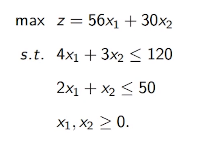使用图解法或者单纯形表方法，可以求得最优解：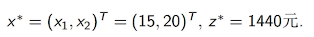对偶问题
现在从另外一个角度来考虑该车间的生产问题，假若有一个个体经营者，手中有一批木器家具生产订单，他想利用该车间的木工与油漆工来完成他的订单，他就要事先考虑付给该车间每个工时的价格
需要考虑的问题：

木器车间觉得有利可图从而愿意替他加工这批订单；
他自己所付时费用总数最小；
设W1为付给木工每个工时的价格，W2为付给油漆工每个工时的价格，则该个体经营者的目标函数每日所付工时总费用最小：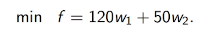但是个体经营者所付的价格不能太低，至少不能低于该车间生产木门、木窗时候所得到的收入，否则该车间觉得无利可图就不会替他加工这批订单，因此w1、W2取值应该满足：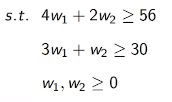那么经过上面的问题梳理，我们把两个问题放在一起看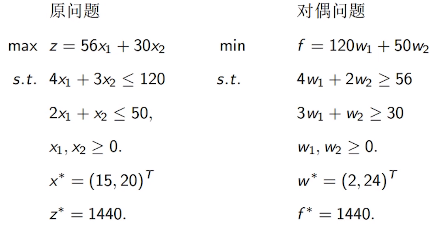左边是车间老板的角度来看，右边的对偶问题是从个体经营者来看，一个求最大值，另外一个求最小值，我们来看一下原问题的max 式子的系数，在右边的对偶问题变成了 下面约束条件的值，而左边的约束条件的值变成了右边对偶问题的min式子的系数

从最后的结果可以看到，虽然最优解的结果不一样，但是最这个最优解的框架下，相应的目标函数的结果都是1440，说明这个订单一定是成功的完成了
转换到一般情况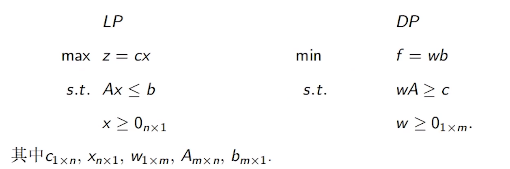LP我们定义就是原问题，DP是对偶问题，Max和Min的式子的系数称之为价值系数，下面的公式称之为约束条件
可以看出：

原问题的价值系数在对偶问题提中成为约束条件的右端项，而原问题的约束条件的右端项在对偶问题成了价值系数；
原问题中约束不等式左端的决策变量的系数，在对偶问题中成为对偶问题决策变W1和W2的系数列向量P1，P2；
原问题中Xi的系数列向量Pi，在对偶问题中就是第i个约束不等式做端对偶变量前的系数

如何迅速的从一个线性规划问题提出对偶问题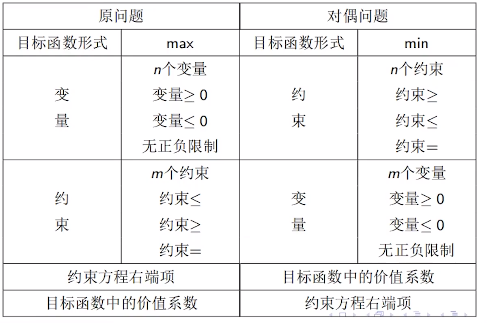写出对偶问题的例子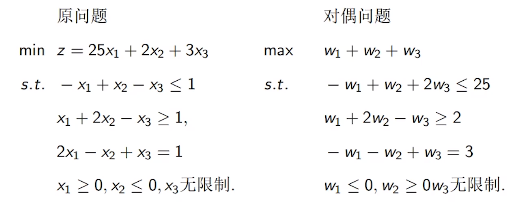展开全文线性规划 机器学习 支持向量机
• 本文主要探讨优化问题中强、弱对偶性以及KKT条件的证明。
目录1.原问题2.对偶问题2.1弱对偶性的一般证明2.2弱对偶性的几何证明2.3强对偶性的几何表示以及条件2.4 slater condition3.KKT条件的证明3.1可行条件3.2互补松弛条件3.3偏导为0条件
1.原问题
首先给出问题的一般形式：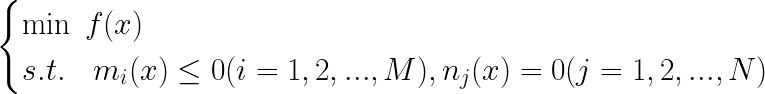上式表明我们一共有M+N个约束条件，对于不是求最小值或者约束条件大于等于0的情况，我们添加一个负号就可以变成上面这种形式。
上述问题我们一般称之为带约束的原问题。
利用拉格朗日乘子法，我们构造一个新的函数以及约束条件如下：其中：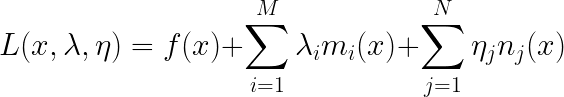我们称上面的问题为无约束的原问题（对x不再有约束）。上述L是拉格朗日乘子法的基本形式，这个就不再证明。
2.对偶问题
对于无约束的原问题，我们先直接给出它的对偶问题形式（其实就是简单交换min和max）：上述问题我们称之为原问题的对偶问题。
2.1弱对偶性的一般证明
所谓弱对偶性，指的是：在再谈SVM（hard-margin和soft-margin详细推导、KKT条件、核技巧）中，我们大致口头证明了弱对偶性的成立，即“凤尾”>=“鸡头”。何谓“凤尾”？我先选出最强的一批人($\max f$)，然后组成实验班，实验班倒数第一就是$\min \ \max f$；何谓“鸡头”？我先选出最弱的一批人($\min f$)，然后在这批比较弱的人当中选出最强的那个人，也即是$\max \ \min f$，那么“鸡头”与“凤尾”孰强孰弱，是显而易见的。
现在我们利用数学推导来大致证明一下弱对偶性。
对于$L(x,\lambda,\eta)$这个函数，我们知道下面这个不等式一定成立：上面这个不等式很好理解，中间$L(x,\lambda,\eta)$我们可以理解为L的值域，值域里面的任何一个数，必然是大于等于它的最小值，小于等于它的最大值。其实这一步已经证明出弱对偶性了，不过为了更容易理解，我们可以进一步说明。
上述不等式最左边的表达式最后是关于$\lambda,\eta$的一个函数，而最右边是一个关于$x$的函数，因此我们又令：因此我们有：证毕。
2.2弱对偶性的几何证明
为了使问题简化，同时方便证明，我们去掉原问题中等式的约束条件，同时不等式约束条件只保留一个，即原问题变成：那么拉格朗日函数就变成：我们又令：即$p^*$是原问题的最优解，$d^*$是对偶问题的最优解。证明弱对偶性实际上就是证明$d^*\leq p^*$。
我们令区域G的表达形式为：D是原问题的定义域，G表示一个个点的集合，点的横坐标是约束条件$u=m_{1}(x)$，纵坐标是原函数$t=f(x)$。
有了上述集合G的定义之后，我们就可以对$p^*,d^*$进行变式。
首先对$p^*$进行变式：因为$t=f(x)$，所以$p^*$实际上就是t的最小值，反映到集合G中去就是指一个点的纵坐标，这个点要满足两个条件：一是肯定要在G中，二是$m_{1}(x)\leq0$也就是该点的横坐标小于等于0。
如下图所示：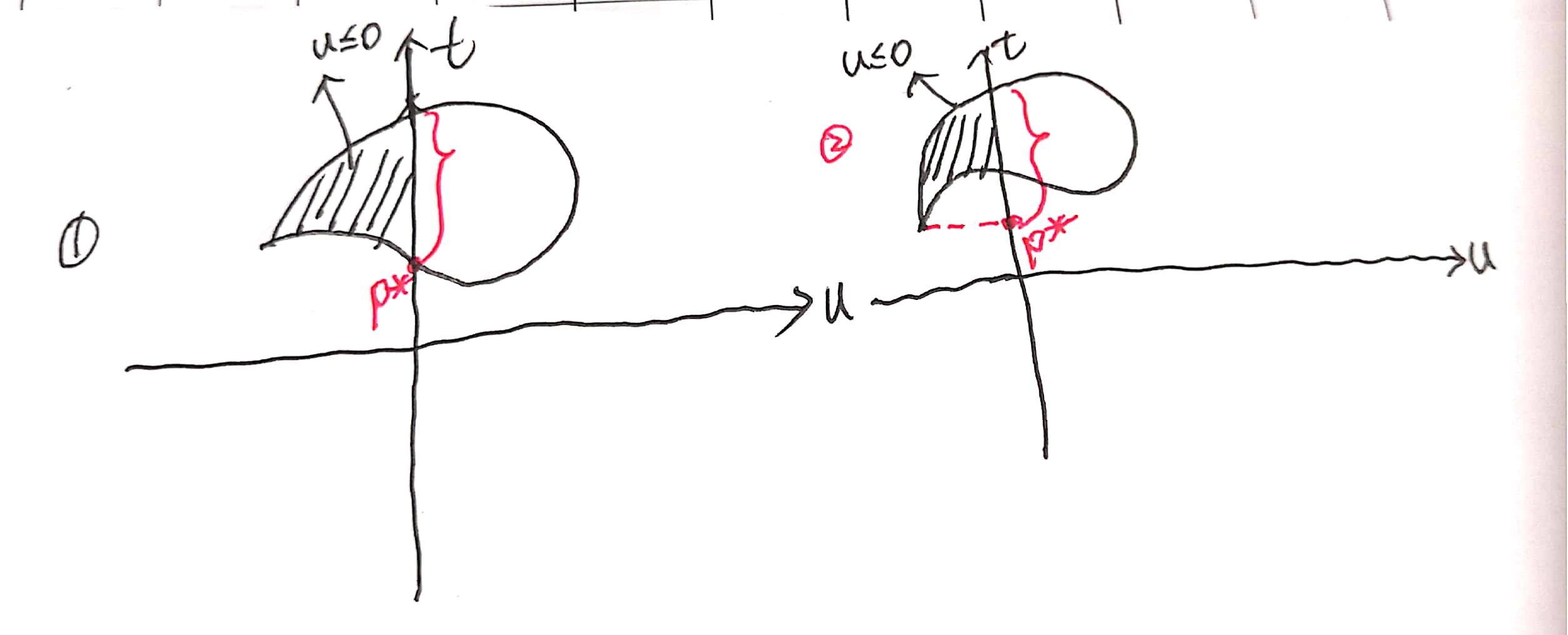我们对$u\leq0$那部分，也就是图中阴影部分上的每个点，找到一个最低的点，它的纵坐标就是$p^*$。
接着对$d^*$进行变形：上述$t+\lambda u$的来源为：对变形后$d^*$我们令：我们先找到$g(\lambda)$在图中的位置：我们知道$t+\lambda u$实际上也是一个值，我们不妨令$t+\lambda u=k$，该式表示一条斜率为$-\lambda$并过(0,k)的直线，我们要找的是$t+\lambda u$的最小值，实际上就是k的最小值，实际上就是该直线与纵轴交点的最小值。而在求$\min \limits_{x}\ t+\lambda u$的最小值时，$\lambda$是固定的，因此斜率$-\lambda$也是固定的：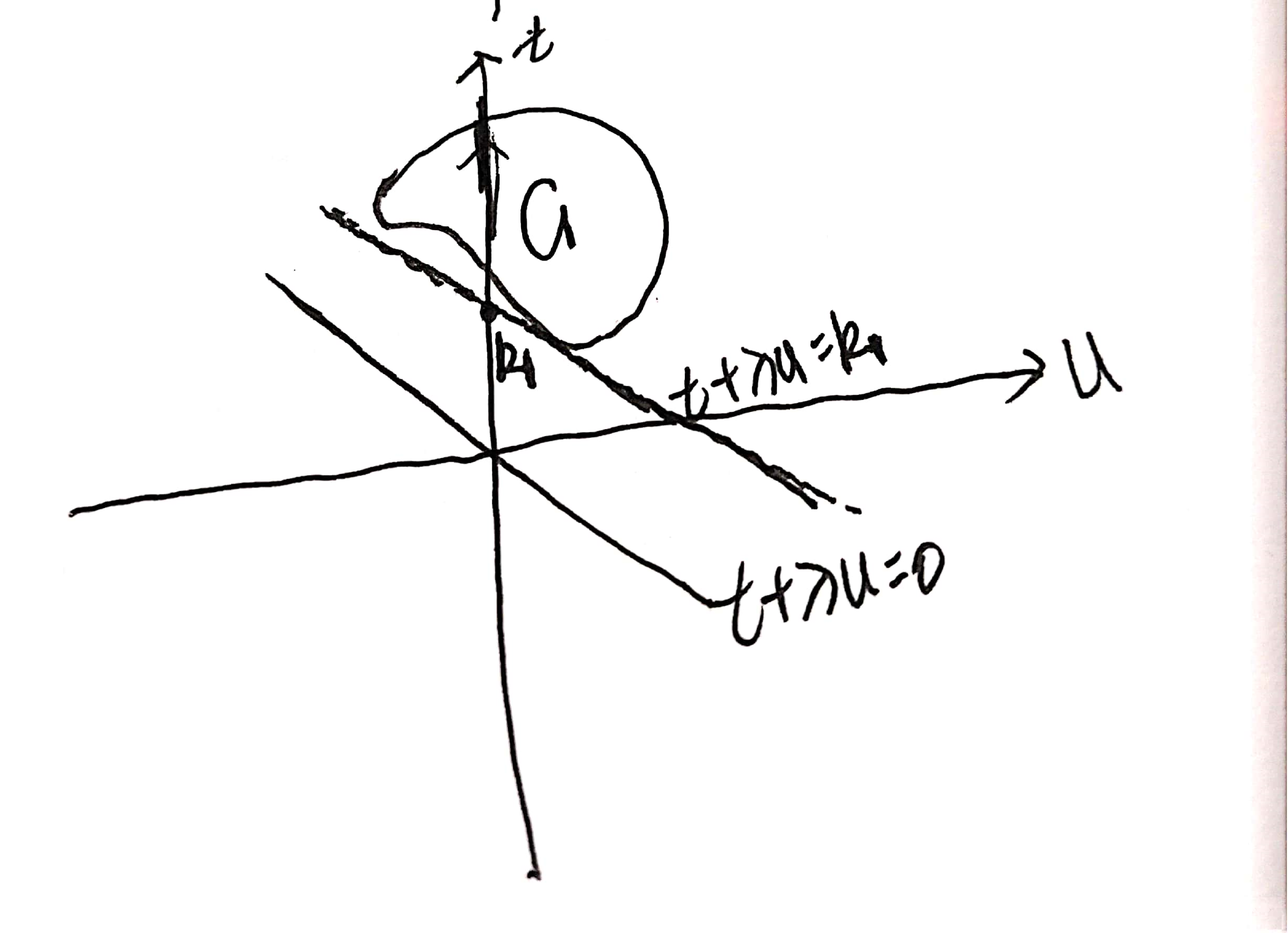我们保持斜率不变移动直线，不断往上移动，则该直线与纵轴交点的纵坐标k也不断增大，因为限制条件还有一个就是$(u,t)\in G$，因此该直线必须经过区域G，我们一直往上移动，直到直线第一次与G相交，记下相应的k值为$k_{1}$，再继续往上也都满足条件，知道该直线与G不再相交，但是现在我们求得是最小值，那么最小值其实就是$k_{1}$，即$g(\lambda)$就等于$k_{1}$。
进一步，我们要求：这里要重点注意：上一步我们求得了$g(\lambda)$就等于$k_{1}$，但是这种情况只是一种情况，在上一步求$g(\lambda)$时，我们假若改变斜率$-\lambda$，那么$k_{1}$的值是会变的，如下所示：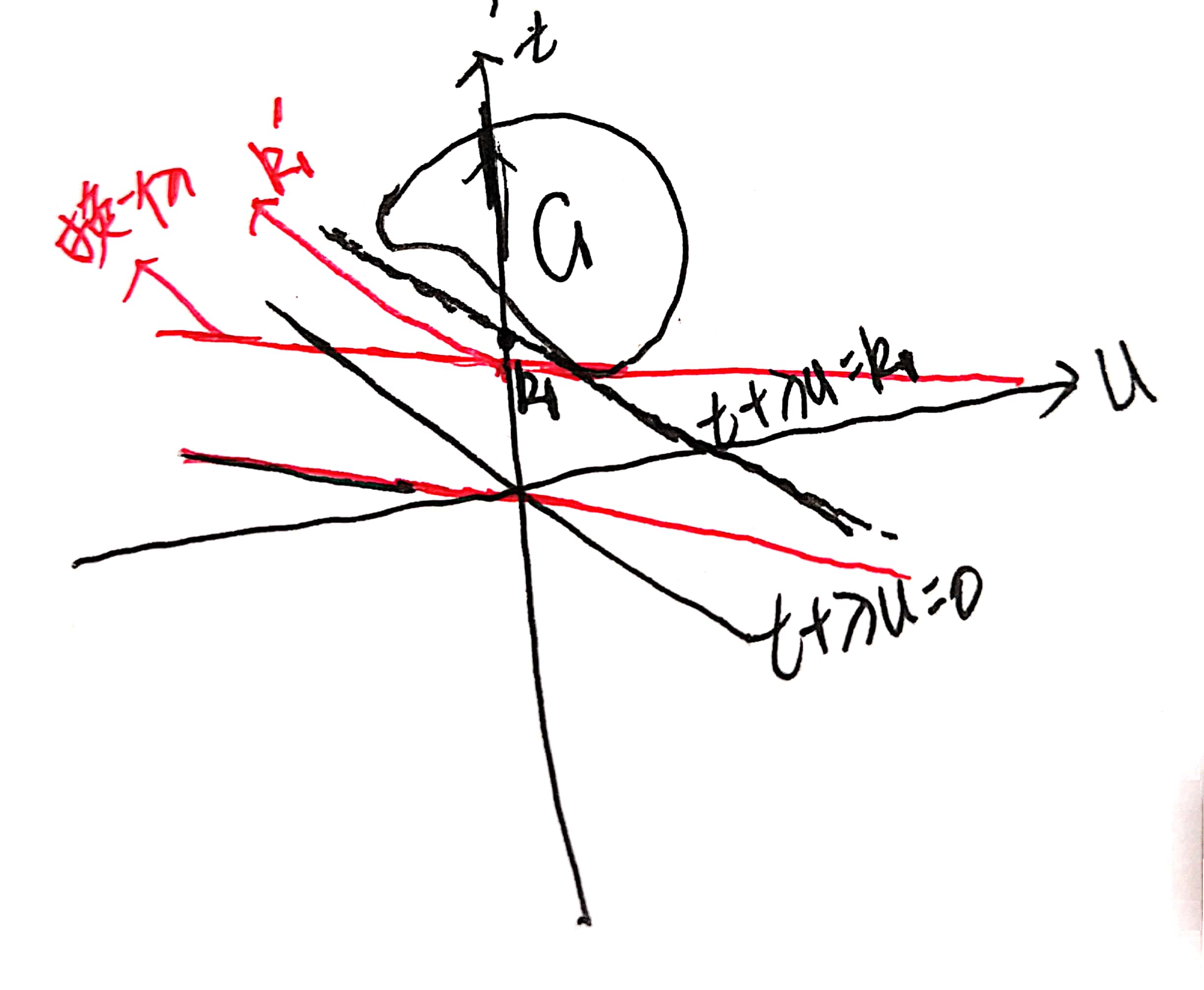我们换一个$\lambda$固定时，$g(\lambda)$也就是$k_{1}$自然也就在变。
第二步要干的其实就是让我们求这个$g(\lambda)$的最大值。那什么时候是最大的？实际上就是以G的最低点为轴，我们旋转直线，直到与左上方最低点相交时，$g(\lambda)$是最大的。如下所示：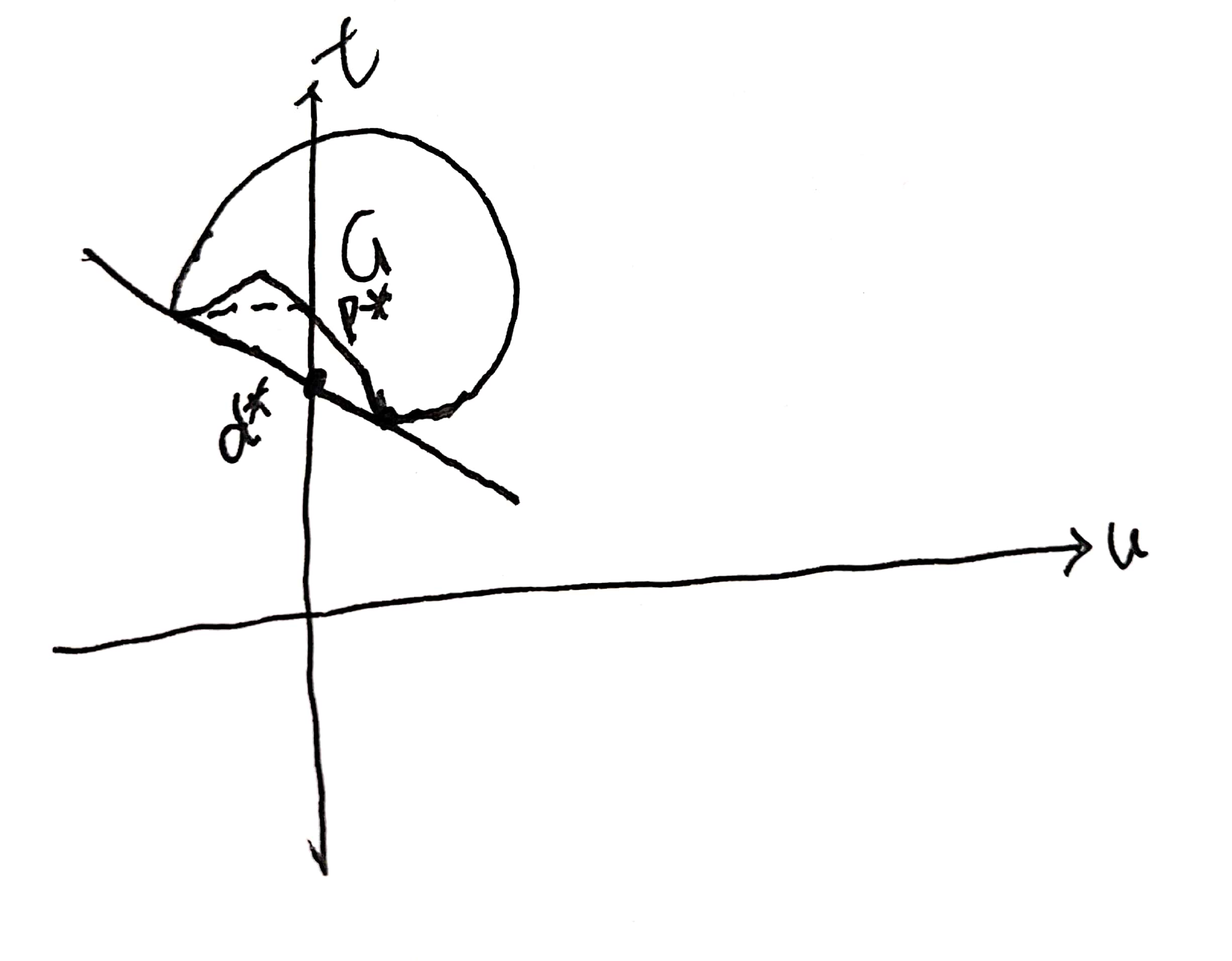可能很多人就有疑问了：我为什么不可以让斜率继续增大？让直线穿过G？这里有疑问的同学不妨回忆一下第一个步骤：我们在确定$g(\lambda)$的时候，第一次相切我们就停止了，而后改变斜率继续相切：如上图所示，假如你继续增大斜率，那么该直线就不跟G的最低点相切了。我们是先推第一步再推第二步，而推第二步的时候肯定必须满足第一步的条件，所以$d^*$只能是在那个位置。从上图也可以看出来：$d^*\leq p^*$，也就是对偶问题的解是小于等于原问题的解的，也即是说满足弱对偶性。因此这里我们进一步证明了弱对偶性。
2.3强对偶性的几何表示以及条件
什么是强对偶性？就是指原问题的解与对偶问题的解是相同的，也即是：$d^*=p^*$。
画个图：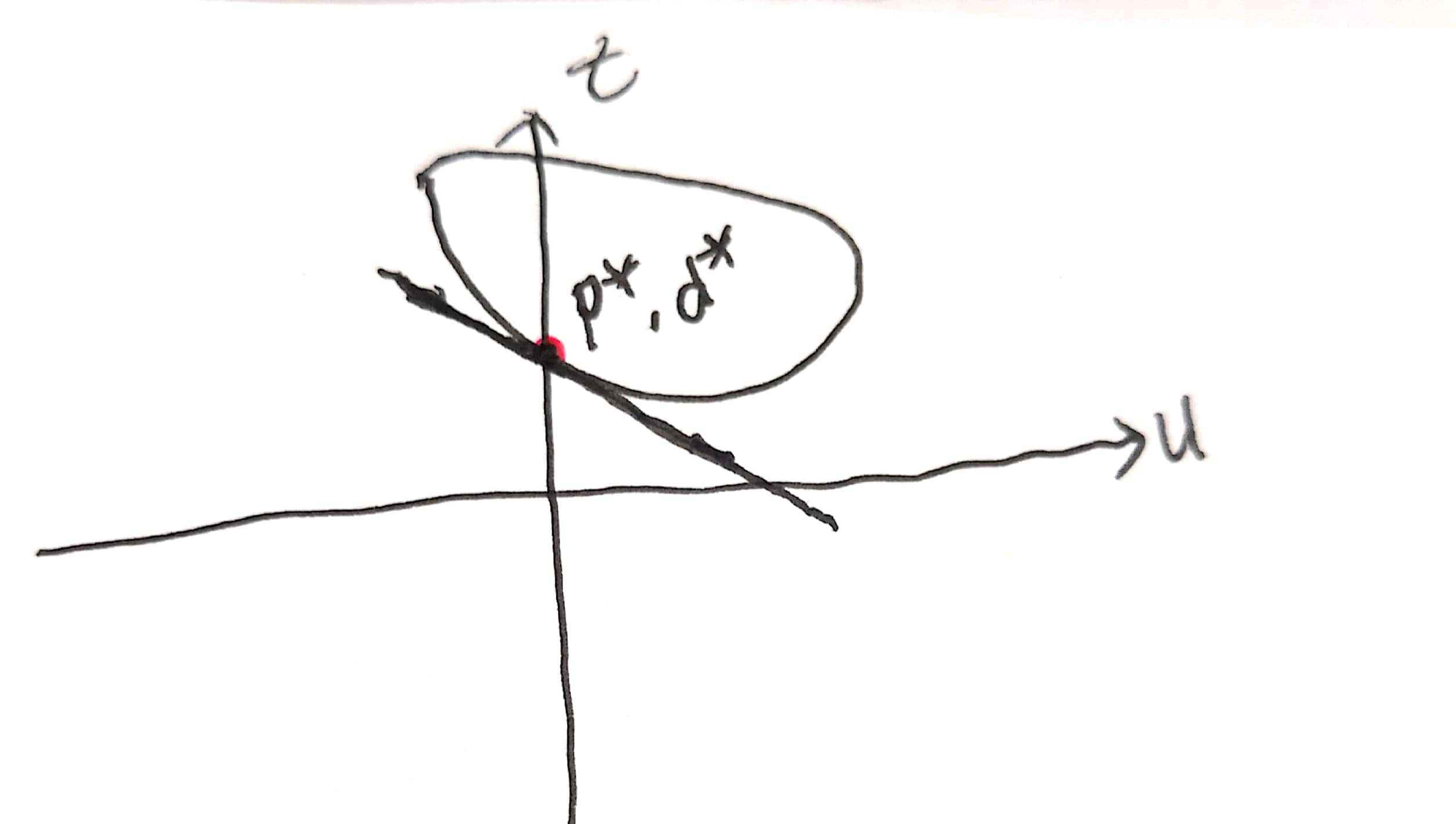假设G是一个凸集，那么根据上面找$d^*$和$p^*$的思路，我们很容易知道这个时候二者是相等的，也就是满足强对偶关系。
那上面这句话的意思就是说：只要是凸集就一定满足强对偶关系。这句话不是正确的，不是所有的凸集都满足强对偶关系，但是加上slater条件就一定满足。
2.4 slater condition
先直接给出slater条件的定义：对于x的定义域D，如果它存在一个内点（不是边界上的点）$x^*$满足对任意的$m_{i}(x)\lt0,i=1,2,...,M$，则说明该问题满足slater条件。
对slater条件做两点说明：

对于大多数的凸优化问题来说，slater condition都是成立的
放松的slater条件：如果约束函数$m_{i}(x),i=1,2,...,M$中有K个是仿射函数，则我们只需要那M-K个约束函数满足第一个条件，我们也说该问题满足slater条件。
什么是仿射函数？仿射函数，即最高次数为1的多项式函数。常数项为零的仿射函数称为线性函数。简单来说，就是比较简单的函数。

对第一个条件进一步说明：为什么我们要满足这个条件？第一个条件放在G中的意思就是：G在u<0部分必须有点存在：假设纵坐标左边没有点，那么在我们寻找$g(\lambda)$时，那条直线实际上就会是纵坐标轴。
3.KKT条件的证明
通过上面的推导我们知道了：满足强对偶关系之后我们就得到一个结论：$d^*=p^*$，但是也到此为止了，我们肯定得解出那些未知最优参数（带 * 的变量），KKT条件就是干这件事。
KKT条件有三部分：可行条件、互补松弛条件以及偏导为0条件，我们一个一个推导。
3.1可行条件
所谓可行条件，指的是一开始就满足的一些条件：这三个条件肯定得满足，这个没啥可说的，天然满足。
3.2互补松弛条件
我们知道：带星号的都是最优解的意思。根据可行条件我们知道：$\lambda_{i}\geq0,m_{i}\leq0$，所以$\lambda_{i}m_{i}\leq0$，所以上面继续变换：因为最后一步等于第一步，所以中间推导步骤中的$\leq$都应该变成=，倒数第三步等于倒数第二步，所以我们有：而前面我们又知道$\lambda_{i}m_{i}\leq0$，所以互补松弛条件如下：3.3偏导为0条件继续看这个推导，第二、三步之间应该用等号连接，即：意思就是说L在$x=x^*$处有最小值，于是偏导为0条件就出来了：于是KKT条件为：证毕。


展开全文kkt条件
• 线性规划的对偶问题对偶问题是原问题
原对偶问题
$\max_y\;b^Ty\\s.t.\;A^Ty+s=c\\s\geq 0\tag{2}$
$A \in \R^{m\times n}, s \in \R^{n}, y \in \R^{m}$
等价问题：
$\min_y\;-b^Ty\\s.t.\;A^Ty+s=c\\s\geq 0\tag{2}$
对偶问题的推导参见博文《线性规划——对偶问题的推导》。

引入拉格朗日函数：$L(x,y,s) = -b^Ty +x^T(A^Ty+s-c)$
$g(x) \triangleq \inf_{y,s}L(x,y,s) \\= \inf_{y,s} \{-c^Tx +(Ax-b)^Ty +x^Ts\} \\=-c^Tx +\inf_y(A^Tx-b)^Ty +\inf_sx^Ts$
分开来看，
$\inf_y(Ax-b)^Ty \\=\left\{ \begin{array}{lr} 0, \;\;\;\;\;\;\;\;\;\;\;Ax-b=0& \\ -\infty, \;\;\;\;\;\;\;otherwise& \end{array} \right.$
$\inf_sx^Ts \\=\left\{ \begin{array}{lr} 0, \;\;\;\;\;\;\;\;\;\;\;z \geq0& \\ -\infty, \;\;\;\;\;\;\;otherwise& \end{array} \right.$
显然，最大化 $g(x)$ 只有在其有下界时有意义。因此得到约束条件：$Ax-b=0, z \geq0。$
原对偶问题的对偶问题为：$maximize \;\;-c^Tx \\s.t.\;\;Ax-b=0\\z \geq0$等价于$minimize \;\;c^Tx \\s.t.\;\;Ax-b=0\\z \geq0$即为线性规划的标准形式。
得出结论： 线性规划的对偶问题的对偶问题是原问题。


展开全文线性规划 推导
• 对偶问题、KKT条件、线性规划中的对偶问题拉格朗日函数和对偶问题强对偶性和KKT条件对偶理论在线性规划里的应用 这篇文章是对pluski文章和Kevin_Duan 文章的整合。第一篇文章主要介绍了如何从拉格朗日函数出发，构造...
一般对偶问题、KKT条件、线性规划对偶问题1. 拉格朗日函数和对偶问题1.1 拉格朗日函数1.2 原问题1.3 对偶问题2. 强对偶性和KKT条件3. 线性规划对偶问题
这篇文章是对pluski和Kevin_Duan 的读后感。看完这两位的文章后，对线性规划对偶问题的来龙去脉有了更深的理解。
这是我CSDN上的第一篇分享，肯定不会是最后一篇，希望能激励自己好好学习，赚钱养猫 ~
1. 拉格朗日函数和对偶问题
1.1 拉格朗日函数
优化问题一般都能转化为如下的形式：
\begin{aligned} \min \quad & f(x)\\ s.t. \quad & h_i(x)\leq0, i=1,...,m\\ & g_i(x)=0, i=1,...,n \end{aligned}   这里面要求$g_i(x)$是仿射的，也就是形如$g_i(x)=Ax+b$.
那么对应的拉格朗日函数是：
$L(x,\lambda,v)=f(x)+\sum_{i=1}^m\lambda_ih_i(x)+\sum_{i=1}^n v_ig_i(x)$
其中 $\lambda_i\geq0$.
这里面$\lambda_i,v_i$都是向量，它们的维度分别由$h_i(x),g_i(x)$确定，反正就是要保证矩阵相乘之后，$\lambda_ih_i(x),v_ig_i(x)$ 都是一个数。 比方说，如果$g_i(x)$ 将 $x$ 映射成一个 $p×1$ 的向量的话， 那么$v_i$ 就是一个 $1×p$ 的向量。
可以看到拉格朗日函数把约束和目标函数融合在了一起。那为什么要引入拉格朗日函数呢？ 神奇的地方就在于，从拉格朗日函数出发，我们能构造出原问题和对偶问题。
1.2 原问题
首先来看看
\begin{aligned} Z(x)=&\max \limits_{\lambda\geq0,v}L(x,\lambda,v)\\ =& \max \limits_{\lambda\geq0,v}f(x)+\sum_{i=1}^m\lambda_ih_i(x)+\sum_{i=1}^n v_ig_i(x) \end{aligned} 这一小节想说的事情就是： $\min Z(x)$ 的最优解 $x^*$，其实是原问题的最优解。
首先，如果$x^*$ 是 $\min Z(x)$ 的最优解，那肯定有 $h_i(x^*)\leq0$ 和 $g_i(x^*)=0$, 不然的话，通过选取合适的 $\lambda$ 和 $v$ ，肯定能让 $Z(x)=\max \limits_{\lambda\geq0,v}f(x)+\sum_{i=1}^m\lambda_ih_i(x)+\sum_{i=1}^n v_ig_i(x)=\infty$ 这样一来 $x^*$ 起码是原问题的一个可行点。
然后，固定 $x=x^*$, 在已知  $h_i(x^*)\leq0$ 和 $g_i(x^*)=0$ 的情况下，为了让 $L(x^*,\lambda,v)$ 最大化，我们会令 $\lambda=0$ (因为 $\lambda\geq0$, $\lambda_ih_i(x)$ 最大的值是0 ). 这样一来，
$Z(x^*)=\max \limits_{\lambda\geq0,v}f(x^*)+\sum_{i=1}^m\lambda_ih_i(x^*)+\sum_{i=1}^n v_ig_i(x^*)=f(x^*)$
也就是说 $x^*$ 也同样让 $f(x)$ 最小了，所以也是原问题的最优解。
总结一下，这一小节就说明了原问题等价于：
$\min Z(x)$
1.3 对偶问题
我们再来看看
$G(\lambda, v)=\min \limits_{x}L(x,\lambda,v)$这一小节想说的事情就是： $\max\limits_{\lambda\geq0,v} G(\lambda, v)$ 是原问题的对偶问题。
对偶问题这个概念有点抽象，其实我们想说明的是，$G(\lambda, v)$充当了原问题的一个下界：
$\max\limits_{\lambda\geq0,v} G(\lambda, v)\leq \min \limits_x Z(x)$ 直白点说，无论取什么 $\lambda$ 和 $v$， $G(\lambda, v)$里面最大的那个，都要比 $Z(x)$ 里面最小的那个小。
设 $(\lambda^*, v^*)$ 和 $x^*$ 分别是对偶问题和原问题的最优解，那么
\begin{aligned} G(\lambda^*, v^*)&=\min \limits_{x}L(x,\lambda^*,v^*)\\ &\leq L(x^*,\lambda^*,v^*)\\ &=f(x^*)+\sum_{i=1}^m\lambda_i^*h_i(x^*)+\sum_{i=1}^n v_i^*g_i(x^*)\\ &\leq f(x^*) \quad (\lambda_i^*h_i(x^*)\leq0, g_i(x^*)=0 ) \end{aligned} 可以看到，$G(\lambda, v)$ 确实充当了原问题 $f(x)$ 的一个下界。接下来，一个自然的问题就是，这个下界可以取到吗？
题外话：对偶问题的不只提供了原问题的下界，它自身还有一个很好的性质，凸性。不论原问题如何，对偶问题一定的凸优化问题，详情见 为什么拉格朗日对偶函数一定是凹函数(逐点下确界)。
2. 强对偶性和KKT条件
记对偶问题的最大值为 $d^*=G(\lambda^*, v^*)$ ，原问题的最小值 $p^*=f(x^*)$。  从上节的推导可知， $d^*\leq p^*$一定成立，这个条件被称作弱对偶性, 对任意优化问题都成立。
强对偶性指的是， $d^*= p^*$ 这一条件， 也即对偶问题的最大值和原问题的最小值刚好重合。
这一节主要想讨论下面这个问题：
当强对偶性成立时，原问题的最优解 $x^*$ 和对偶问题的最优解 $(\lambda^*, v^*)$ 会有什么关系呢？（是的，KKT要来了！）
当强对偶性成立， $d^*=G(\lambda^*, v^*)=f(x^*)=p^*$的时候，有
\begin{aligned} f(x^*)= G(\lambda^*, v^*)&=\min \limits_{x}L(x,\lambda^*,v^*)\\ &\leq L(x^*,\lambda^*,v^*)\\ &=f(x^*)+\sum_{i=1}^m\lambda_i^*h_i(x^*)+\sum_{i=1}^n v_i^*g_i(x^*)\\ &\leq f(x^*) \quad \quad(0) \end{aligned} 由于夹逼定理，上面的这两个 $\leq$ 号都是 $=$ 号。
第一个等号告诉我们，$x^*$ 是函数 $L(x, \lambda^*,v^*)$ 的最小值，所以它是 $L(x, \lambda^*,v^*)$ 的某个极小值点。在 $x^*$  处，$L(x, \lambda^*,v^*)$ 关于 $x$ 的梯度等于0：
$\nabla f(x^*)+\sum_{i=1}^m\lambda_i^* \nabla h_i(x^*)+\sum_{i=1}^n v_i^* \nabla g_i(x^*) = 0 \quad(1)$ 第二个等号告诉我们，$\sum_{i=1}^m\lambda_i^*h_i(x^*)=0$ ，而里面每一项都是非正的，所以每一项都等于0：
$\lambda_i^*h_i(x^*)=0 \quad i=1,..., m \quad(2)$
加上原问题及对偶问题可行域的条件：
\begin{aligned} &h_i(x^*)\leq0, i=1,...,m \quad(3)\\ &g_i(x^*)=0, i=1,...,n \quad(4)\\ &\lambda_i^*\geq0, i=1,...,m \quad(5) \end{aligned}
$(1)-(5)$合起来，就是 $x^*$ 和 $(\lambda^*, v^*)$ 共同要满足的条件，是不是很眼熟？ 没错，这就是KKT(Karush–Kuhn–Tucker)条件！
维基百科里，KKT条件的叙述是这样的：“the KKT conditions are the necessary conditions for a solution in nonlinear programming to be optimal, provided that some regularity conditions are satisfied.”
也就是说，在regularity conditions成立的情况下，最优解 $x^*$ 会满足KKT条件 (Necessary conditions)。这里的regularity conditions可以理解成，让强对偶性成立的条件。
顺带一提，如果原问题是凸优化问题（目标函数核约束空间都是凸的），那么令KKT条件成立的 $x^*$ 就是原问题的最优解 (Sufficent conditions)。(答案就在 $(0)$ 式里面)
3. 线性规划对偶问题
最后，我们看看怎么利用一般优化问题的对偶理论，来推导出线性规划的对偶问题 (Dual LP)。
一般来说，线性规划问题都可以转化成下面的形式：
\begin{aligned} \min \quad &C^Tx\\ s.t. \quad &Ax-b=0\\ &x\geq0 \end{aligned} 根据 $1.1$ 章节，这个优化问题对应的拉格朗日函数是：
$L(x,\lambda,v)=C^Tx-\lambda^Tx+v^T(Ax-b), \quad \lambda\geq0$ 稍微对维度解释一下，如果 $A\in R^{m×n}$ 的话（线性规划里有 $m$ 条约束，$n$ 个变量）, 那么 $v \in R^{m×1}$ , $\lambda \in R^{n×1}$。简单来说，就是得让矩阵相乘的结果是一个数。
根据 $1.3$ 章节，从拉格朗日函数出发，对应的对偶问题是：
\begin{aligned} G(\lambda,v) & = \min \limits_{x} L(x,\lambda,v) \\ & = \min \limits_{x} -v^Tb+(C^T-\lambda ^T+v^TA)x\\ &=\begin{cases} -\lambda^Tb, C^T-\lambda ^T+v^TA=0 \\ -\infty, otherwise \end{cases} \end{aligned}为了让问题有意义，$C^T-\lambda ^T+v^TA=0$ 必须成立，所以对偶问题是：
\begin{aligned} \max \quad &G(\lambda,v)= -v^Tb\\ s.t. \quad &C^T-\lambda ^T+v^TA=0\\ &v\geq0 \end{aligned} 把转置翻一下，用 $-v$ 代替 $v$ ，就可以得到下面这个等价的、更常见的 Dual LP：
\begin{aligned} \max \quad &b^Tv\\ s.t. \quad &A^Tv +\lambda =C\\ &\lambda \geq0 \end{aligned} 线性规划问题是满足强对偶性的，所以原问题的最优解 $x^*$ 和对偶问题的最优解 $(\lambda^*, v^*)$ 会满足KKT条件。
其中一条就是
$\lambda^Tx=0，$也就是线性规划里常说的Complementary Slackness。
(原问题里面的某个 $x_i$ 是基变量时，对应的对偶约束一定是binding的，也即 $\lambda_i=0$)
KKT里面梯度为0的条件，其实是对偶问题的约束 $A^Tv +\lambda =C$。


展开全文• 对偶问题任何可行解的目标值都是另一问题最优目标值的界。（推论：原对 偶问题目标值相等的一对可行解是各自的最优解） 强对偶性 原对偶问题只要有一个有最优解，另一个就有最优解，并且最优目标值相等。 对偶问题...线性规划
• 在约束最优化问题中，常常利用拉格朗日对偶性（Lagrange duality）将原始问题转换为对偶问题，通过解对偶问题而得到原始问题的解。 拉格朗日对偶是在拉格朗日乘数法基础之上，通过变换原始问题的求解形式得到的相...算法 最优化方法
• 介绍拉格朗日对偶中的原始问题、对偶问题以及原始问题与对偶问题的关系拉格朗日乘子 支持向量机 最优化方法 拉格朗日乘数法
• ## 线性规划对偶问题

千次阅读 多人点赞 2021-04-28 23:49:31
线性规划对偶问题线性规划及单纯形法〇． 前言一． 对偶问题的提出二． 对称形式下对偶问题的一般形式三． 非对称形式的原-对偶问题关系四． 对偶问题的基本性质五． 对偶问题的单纯形法描述六． 影子价格七． 利用...
• 一、对偶理论 、 1、对称性定理 、 2、弱对偶定理 、 ...二、原问题与对偶问题对应关系 、 二、对偶理论的相关结论 、 1、对偶问题存在 、 2、对偶问题转化 、 3、对偶问题的解 、 4、互补松弛定理最优性定理
• 文章目录如何快速写出对偶问题正常问题，则正常对偶非正常问题，则不正常处理对偶理论对偶单纯型法对偶单纯型法不是求解对偶问题的，而是求原问题的灵敏度分析对于某些数据发生变化时，最优解（最大值或最小值）将...
• =z*），所谓的能否转化成对偶问题主要是指强对偶定理是否成立（即是否有v*=z*）。对线性规划，满足特定regularity条件（一般来说并不难）的半正定规划或凸优化问题也是成立的。 至于一些非凸问题或者整数规划问题，...
• 对偶问题2. 原问题和对偶问题的对应关系3. 举例参考 1. 对偶问题 任一线性规划问题都存在另一与之伴随的线性规划问题，它们组成一对互为对偶的线性规划问题。 线性规划的对偶问题与原问题互为对偶，线性规划的原...线性规划
• 原始问题与对偶问题（转）每一个线性规划问题，我们称之为原始问题，都有一个与之对应的线性规划问题我们称之为对偶问题。原始问题与对偶问题的解是对应的，得出一个问题的解，另一个问题的解也就得到了。并且原始...
• 一、工厂生产产品模型、 二、问题一 : 生产利润最大化、 三、问题二 : 设备出租问题、 四、对偶问题引入、线性规划
• 在线性规划早期发展中最重要的发现就是对偶问题，即每一个线性规划问题(称为原始问题)都有一个与它对应的对偶线性规划问题（称为对偶问题），下图中最后那个是互补松弛定理。 正确的是B，因为一个问题有可行解...
• ## 对偶问题的转换

千次阅读 2019-07-21 10:57:32
对偶问题 下面是博主对对偶问题的一些个人理解，博主也是小白一个，有不当之处欢迎评论指教。 这个是百度里面的解释，是原问题和对偶问题的转变。 例子 小明同学拥有一家工厂，他现在有2种获利途径： ......